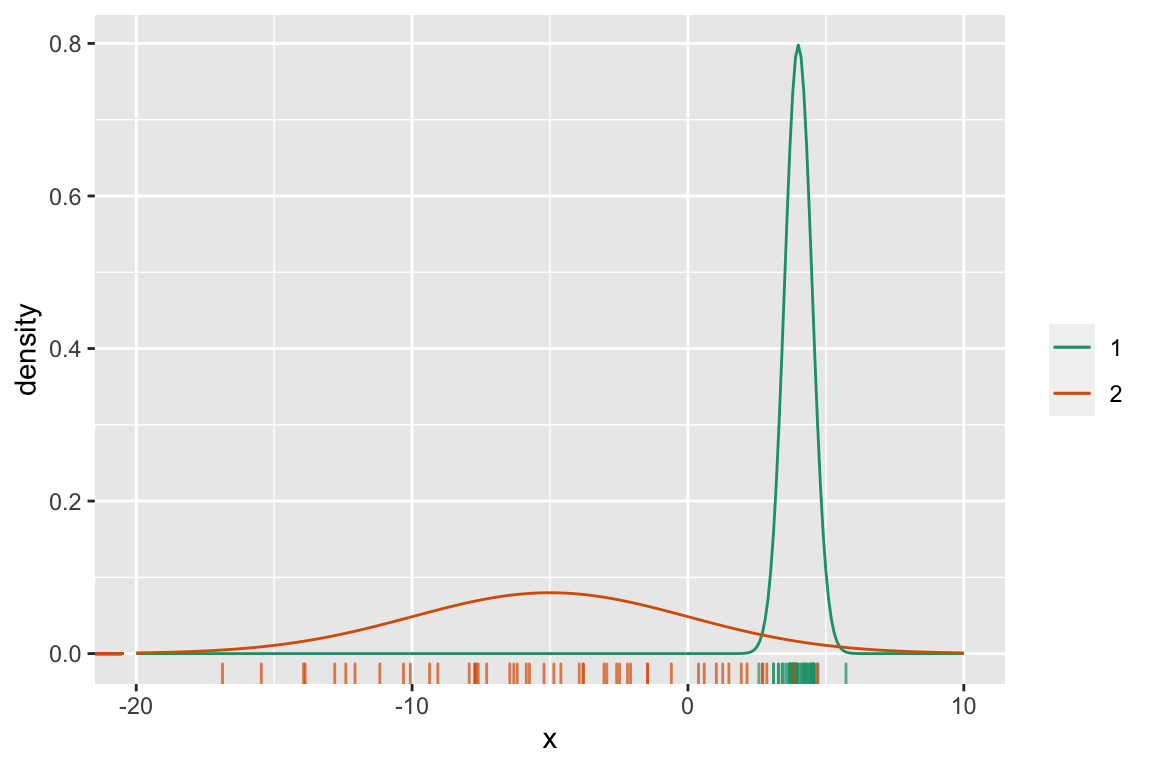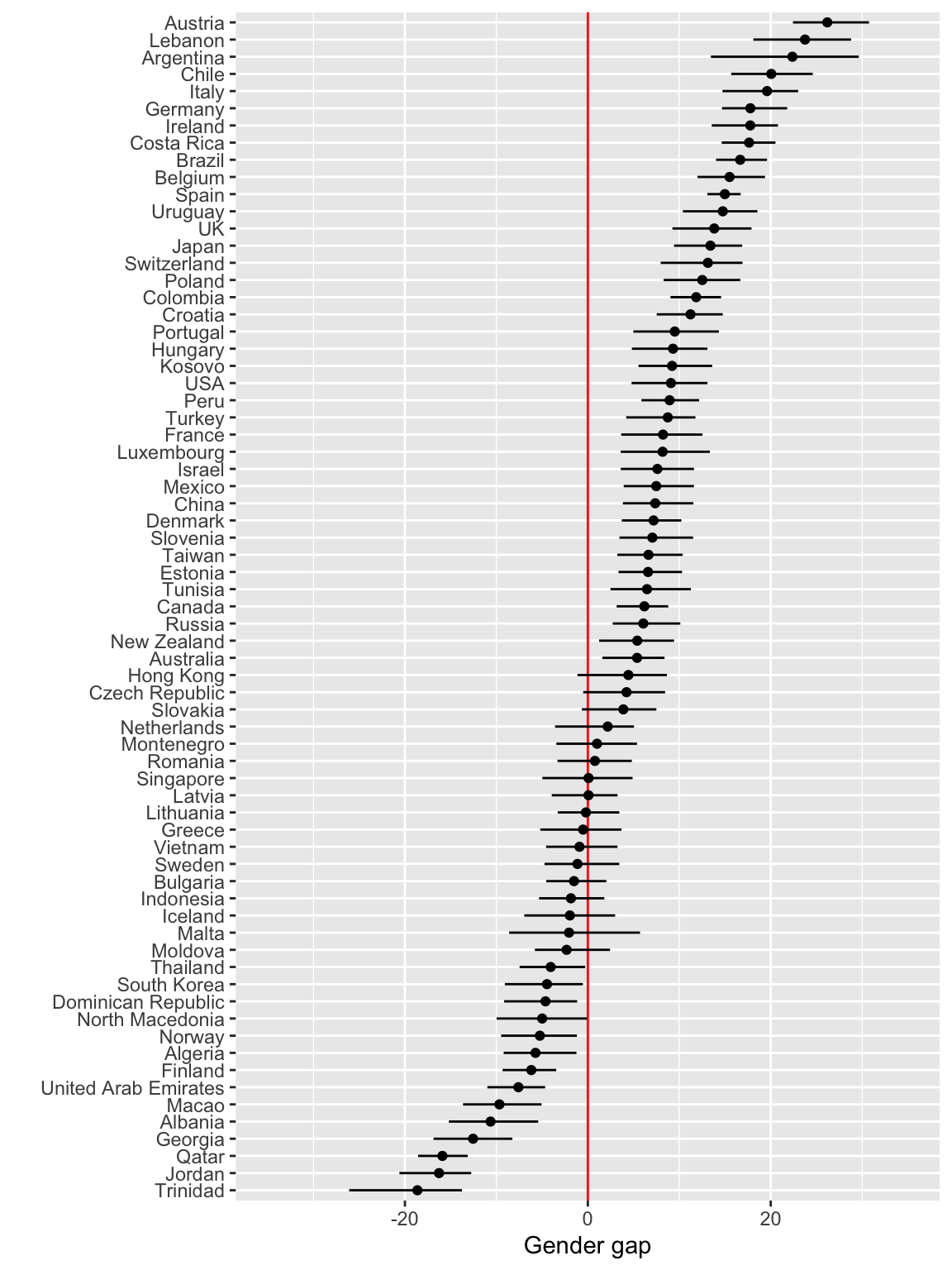## Instructions

• Assignment needs to be turned in as Rmarkdown, and as html, to moodle. That is, two files need to be submitted.
• You need to list your team members on the report. For each of the four assignments, one team member needs to be nominated as the leader, and is responsible for coordinating the efforts of other team members, and submitting the assignment.
• It is strongly recommended that you individually complete the assignment, and then compare your answers and explanations with your team mates. Each student will have the opportunity to report on other team member’s efforts on the assignment, and if a member does not substantially contribute to the team submission they may get a reduced mark, or even a zero mark.
• R code should be hidden in the final report, unless it is specifically requested. Doing equations in Rmarkdown uses latex syntax. It can be fiddly to get it right because small errors corrupt the typesetting. If you have trouble with this feel free to hand-write the math, and include as an image.
• Original work is expected. Any material used from external sources needs to be acknowledged.
• To make it a little easier for you, a skeleton of R code is provided in the Rmd file. Where you see ??? means that something is missing and you will need to fill it in with the appropriate function, argument or operator. You will also need to rearrange the code as necessary to do the calculations needed.

## Marks

• Total mark will be out or 25
• 3 points will be reserved for readability, and appropriate citing of external sources
• 2 points will be reserved for reproducibility, that the report can be re-generated from the submitted Rmarkdown.
• Accuracy and completeness of answers, and clarity of explanations will be the basis for the remaining 20 points.

## Exercises

1. (5pts)This question is about the normal distribution, and how it relates to the classification rule provided by quadratic discriminant analysis.

1. Write down the density function for a univariate normal distribution ($$p=1$$), with mean $$\mu_k$$ and variance $$\sigma_k$$.

2. Show that the quadratic discriminant rule for two groups ($$K=2$$), $$\pi_1=\pi_2$$ is equal to: Assign a new observation $$x_0$$ to group 1 if $-\frac12 \left( \frac{1}{\sigma_1^2}- \frac{1}{\sigma_2^2} \right) {x_0^2} + \left(\frac{\mu_1}{\sigma_1^2}-\frac{\mu_2}{\sigma_2^2}\right)x_0 -\frac12 \left(\frac{\mu_1^2}{\sigma_1^2}- \frac{\mu_2^2}{\sigma_2^2} \right) -\log{\sigma_1}+\log{\sigma_2}>0$

3. Suppose $$\mu_1=4, \mu_2=-5, \sigma_1=0.5, \sigma_2=5$$ simulate a set of 50 observations from each population. Make a plot of the population model, and add these samples as a rug plot on the horizontal axis. (See the lecture notes for a similar plot and code for linear discriminant analysis.)

4. Write down the rule using these parameter values, and sketch the boundary corresponding to the rule on the previous plot.

5. If instead you had made a mistake and assumed that the two variances were equal, this would have produced a linear discrimant rule. Mark this boundary on the previous plot. Explain why and how this differs from result of the QDA rule.

2. (4pts)In this question you are going to practice conducting bootstrap to obtain confidence intervals for a reasonably complicated yet simple analysis.

A significant gender gap in maths performance in favour of male students has returned, despite closing in 2015 Natassia Chrysanthos, Sydney Morning Herald

Last December, the 2018 OECD PISA results were released. These are standardised test scores in math, reading and science, of 15 year olds across the globe. It led to a flurry of articles in the news about slipping standards of Australian students. If you also browsed the news of other countries (including New Zealand, Indonesia, Finland), you would find that many had similarly woeful stories. The above headline focuses on the math gap. To explore this, we will compute bootstrap confidence intervals for the difference between weighted averages for boys and girls in each country. The data is from the 2015 results.

The code block below will compute the difference between weighted averages for boys and girls in each country. A weighted average is often used with survey data, to reflect how the sampling was done relative to the population characteristics. The weighted average will typically better reflect the population mean.

This block of code will compute 90% bootstrap confidence intervals for the weighted mean difference.

This block of code will add country names, and make dotplots with confidence intervals for the math gap for each country.

Write a paragraph explaining what you learn about the math gap across the countries tested in 2015.

1. (9pts)A cross-rate is an exchange rate between two currencies computed by reference to a third currency, usually the US dollar. The data file rates_Nov19_Mar20.csv was extracted from https://openexchangerates.org using the code below (my API key has been hidden from you):
1. (1)What’s the data? Make a plot of the Australian dollar against date. Explain how the Australian dollar has changed relative to the US dollar over the 5 month period.

Over the 5 month period the Australian dollar has weakened against the US dollar, with a big decline in mid-March as the coronavirus impact affected the world.

1. (1)You are going to work with these currencies: AUD, CAD, CHF, CNY, EUR, GBP, INR, JPY, KRW, MXN, NZD, RUB, SEK, SGD, ZAR. List the names of the countries and currency name that these codes refer to. Secondary question: why is the USD a constant 1 in this data.

The US is the base rate, against which all other currencies are compared.

1. (2)The goal of the principal component analysis is to examine the relative movement of this subset of currencies, especially since coronavirus emerged until the end of March. PCA is used to summarise the volatility (variance) in the currencies, relative to each other. To do this you need to:

• Standardise all the currencies, individually. The resulting values will have a mean 0 and standard deviation equal to 1.
• Flip the sigm so that high means the currency strengthened against the USD, and low means that it weakened. Its easier to explain trends, if you don’t need to talk with double-negatives.
• Make a plot of all the currencies to check the result.
2. (5)Conduct a principal component analysis on the subset of currencies. You need to work from a wide format of the data, where dates are in the columns, and currencies are in the rows. Normally, PCA operate on standardised variables but for this data, you need to NOT standardise each date. Think about why this is best.

• Why is this data considered to be high-dimensional?
• Make a scree plot to summarise the variance explained by cumulative principal components. How much of the total variation do two PCs explain?
• Plot the first two principal components. Write a summary of what you learn about the similarity and difference between the curreencies.
• Plot the loadings for PC1. Add a base line set at $$1/\sqrt{15}$$. Why use this as a guide? What time frame generated a big movement (or divergence) in the currencies? Which currencies strengthened relative to the USD in that period? What happened to the Australian dollar? Answer these questions in a paragraph, written in your own words.
• Do the same analysis for PC2. What time frame was there another movement of currencies? Which currencies primarily strengthened, and which weakened during this period?
• Finish with a paragraph summarising what variability the principal components analysis is summarising. What dimension reduction is being done?
1. (2pts)What’s wrong with the following statement?

Principle component analysis is a dimension reduction technique.

### Plots for the different parts will look something like these